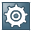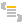Show TOC

###Compression TechniquesIf you want to send large quantities of data, we recommend that you compress it first to keep the transports as small as possible.

#### Example

The example below shows how you might use data compression.Syntax `DATA: html_body TYPE string.` `…` `IF compress NE space.` ` CALL METHOD server->response->set_header_field(` ` name = 'Content-Type' ` ` value = 'text/html' ).` `*` `* is compression supported and does client support gzip` `* compression technique` `*` ` CALL METHOD server->set_compression` ` EXPORTING` ` options = if_http_server=>co_compress_in_all_cases` ` EXCEPTIONS` ` compression_not_possible = 1` ` OTHERS = 2.` ` IF sy-subrc <> 0.` ` CONCATENATE` ` ''` ` ''` ` 'Compress nicht möglich '(001) '
'` ` ''` ` ''` ` INTO html_body.` ` ELSE.` ` CONCATENATE ''` ` ''` ` 'Compress erfolgreich '(001) '
'` ` ''` ` ''` ` INTO html_body.` ` ENDIF.` `*` `* if compression could be activated the body will be transferred` `* as gzip compressed body` `*` ` CALL METHOD server->response->set_cdata( data = html_body ).` ` ENDIF.` ` ` End of the code.